Courses

# Percentile Booster - Quant: Number system CAT Notes | EduRev

## CAT : Percentile Booster - Quant: Number system CAT Notes | EduRev

The document Percentile Booster - Quant: Number system CAT Notes | EduRev is a part of CAT category.
All you need of CAT at this link: CAT

1. What two-digit number is less than the sum of the square of its digits by 11 and exceeds their doubled product by 5?
(a) 15, 95
(b) 95
(c) Both (a) and (b)
(d) 15, 95 and 12345
Correct option: A
Solution:
Of course with the options here you can check the values directly to see that the required condition fits for the numbers 15 and 95, respectively. However, in case you were solving this without options, and you were required to find the two digit numbers that satisfied the given condition, you would need a completely different process to solve this.

2. Find the lower of the two successive natural numbers if the square of the sum of those numbers exceeds the sum of their squares by 112.
(a) 6
(b) 7
(c) 8
(d) 9
Correct option: B
Solution:
Again, spotting this with options is quite easy as we can see that 72 + 82 = 113 and that is 112 less than the value of (7 + 8)2 = 225. Without options here you can think of a2 + b2 + 112 = (a + b)2 →2ab = 112 or ab = 56. Since, the numbers are consecutive, sifting through the factor pairs of 56 we can see the numbers as 7 & 8, respectively.

3. In a four-digit number, the sum of the digits in the thousands, hundreds and tens is equal to 14, and the sum of the digits in the units, tens and hundreds is equal to 15. Among all the numbers satisfying these conditions, find the number the sum of the squares of whose digits is the greatest.
(a) 2572
(b) 1863
(c) 2573
(d) None of these
Correct option:  D
Solution:
The required number can be formed by making the hundreds and the tens digits as small as possible. You would also need to make the middle digits equal to each other (or as equal to each other as possible) 8339 would be the number required.

4. A four digit number wxyz is such that x + y = 2w & y + 6z = 2(w + x) & w + 5z = 2y. Find the sum of such four digit numbers which satisfy the given conditions.
Solution:
x + y = 2w ……1 y + 6z = 2(w + x) …….2 w+ 5z = 2y…..3 From equation 2 – equation 1 we get: 6z – x = 2x or x = 2z Substituting x = 2z in equation 1, we get : 2w − y= 2z …..(4) Solving equation 3 and 4 we get w = 3z & y = 4z z: y: x: w = 1: 4: 2: 3 So 3241 & 6482 are two possible values of wxyz. So the required sum = 3241+ 6482 = 9723. Alternately, once you have the relationship x = 2z, you can think of values and try to fit in the conditions of the other equations. z and x can take only 4 feasible values: viz 1,2; 2,4;3,6 & 4,8. This gives us four possibilities for the numbers: _2_1; _4_2; _6_3; _8_4. The fourth of these, with z = 4 can be eliminated by looking at the third equation (w + 5z = 2y) as it would need y to be greater than 10. For z = 3 & x = 6; we get w = 1 & y = 8 or w = 3 and y = 9 from the third equation. Both these values do not match the second equation. For z = 2 & x = 4; we get w = 2 & y = 6 or w = 4 and y = 7 or w = 6 and y = 8 or w = 8 and y = 9. Checking for the second equation, only the values of w = 6 & y = 8 matches. Hence, we get the number 6482. Likewise, when you check for z = 1 & x = 2, you would be able to find the number 3241.

5. X is a number formed by writing the first 1002 natural numbers one after another from left to right then find the remainder when X is divided by 9 is
Solution:
Remainder when X is divided by 9, is same as remainder when sum of digits of X is divided by 9. Sum of digit of first 999 natural numbers is 13500, which is divisible by 9. Now sum of digits of 1000, 1001, 1002 are 1, 2 and 3, respectively. Sum of digits of X is 9n + (1 + 2 + 3) = 9n + 6. So the required remainder is 6.

6. What is the maximum number of elements that one can pick from the set of natural numbers from 1 to 20 such that the product of no two of them results in a perfect square or perfect cube?
Solution:
First of all pick all the prime number i.e. {2, 3, 5, 7, 11, 13, 17, 19}. We cannot pick perfect square numbers i.e. 4, 9, 16 & perfect cube numbers i.e. 8. Now we are left with the numbers 6, 10, 12, 14, 15, 18, 20 out of which 12 & 18 will give perfect square numbers when we multiplied them with 3 and 2, respectively. Also, we would need to take 1 into this list. So we have a total 14 such numbers {1, 2, 3, 5, 6, 7, 10, 11, 13, 14, 15, 17, 19, 20}. Note: If you try to improve this solution, by taking the perfect squares and the perfect cubes in, you would first need to get rid of 1, in the list. Also, you can take only one perfect square number (as if you were to take two perfect squares, their product would be a perfect square too). With respect to the perfect cube 8, we can see that 8 × 2 = 16 is a perfect square. Hence, if we try to take in 8, we would need to remove 2 from our list. Thus, you can see that you can take this list to a maximum of 14 numbers

7. Find the sum of all odd three-digit numbers that are divisible by 5.
(a) 50,500
(b) 50,250
(c) 50,000
(d) 49,500
Correct option: D
Solution:
The required numbers would be numbers in the Arithmetic Progression 105,115,125,….995. The sum of this series would be given as n × Average = 90 × 1100 /2 = 49500

8. The product of a two-digit number by a number consisting of the same digits written in the reverse order is equal to 2430. Find the lower number.
(a) 54
(b) 52
(c) 63
(d) 45

Correct option: D

Solution:
A factor pair search of 2430 would give you the answer as 45 × 54. Hence, 45 is the correct answer.

9. Find the two-digit number the quotient of whose division by the product of its digits is equal to 8/3, and the difference between the required number and the number consisting of the same digits written in the reverse order is 18?
(a) 86
(b) 42
(c) 75
(d) None of these
Correct option: D
Solution:
The second condition requires the number to be one of 31, 42, 53, 64, 75, 86 or 97. 64 is the only number amongst these that meets the requirement of the quotient of the number divided by its’ product of digits is 8/3. However, it is not given in the options. Hence, the correct answer is none of these.

10. If ‘a’ is a natural number and HCF of a, a + 5 is 5. If the LCM of the two numbers is a three-digit number, then what is the difference between the maximum & minimum possible values of the smaller number?
(a) 25
(b) 35
(c) 40
(d) 45
Correct option: D
Solution:
Let a = 5k then a + 5 = 5(k + 1). Both k, k + 1 are co-prime. LCM of a, a + 5 = 5.k.(k + 1) For k = 4, 5k(k + 1)=100. So minimum possible value of smaller number is 20. Maximum value of k for which the LCM is a threedigit number is 13. Maximum possible value of the smaller number = 65 So the required difference = 65 − 20 = 45.

11. How many numbers less than 100 have exactly four factors?
Solution:
Since both the numbers are odd. there are no 2's in their prime factors. Since, their HCF is 225, both these numbers would necessarily have 32 x 52 inside them From the information, that both these numbers have 36 factors, we can realize that 36 factors can only occur in eases where the prime factors of the numbers look as follows: p8 x q3-; p11 x q2- ; p2 : x q3 x r2 & p2 x q2 x r x s . Amongst these, the best strategy to make smaller numbers satisfying the criteria would obviously be to use the structures: p2 x q3 x r2 and p2 x q2 x r x s. Since, the third prime factor of the two numbers cannot be the same (else it would change the HCF), we would need to introduce 7 and 11.

Also, we would not try to increase the powers of 7 and 11 as they are comparatively larger as compared to 3 and 5. Thus, we can visualize the numbers: 32 x 52 x 11 x 13, 33 x 52 x 72. The required smallest LCM w ould be 33 x 52 x 72 x 11 x 13.

12. Two odd numbers have 36 factors each and the HCF of these two numbers is 225. What is the minimum possible LCM of these two numbers if the power of any prime factor in these two numbers is not more than 3?
Solution:

7! = 5040.

When we divide 7! By 17 it leaves a remainder 8.

When [(7!)6!]¹⁷⁷⁷⁷ is divided by 17 it leaves a remainder that is same as when divided [(8)6!]¹⁷⁷⁷⁷ or [(8)⁷²⁰]¹⁷⁷⁷⁷ or [(16)⁵⁴⁰]¹⁷⁷⁷⁷ by 17.

The remainder when [(16)⁵⁴⁰]¹⁷⁷⁷⁷ or [(17 - 1)⁵⁴⁰]¹⁷⁷⁷⁷ divided by 17 is 1 (since power of 16 is even).

13. The remainder obtained when 43101 + 23101 is divided by 66 is
(a) 2
(b) 10
(c) 5
(d) 0
Correct option: D
Solution:

43101 - 23101 is of the form an + bn with n odd Such a number can be written to be a multiple of (a - b).

Thus, the given expression is a multiple of (43 + 23)= 66. Hence, the required remainder would be 0.

14. The last three-digits of the multiplication 12345 x 54321 will be
(a) 865

(b) 745
(c) 845
(d) 945
Correct option: B
Solution:

Divide the given expression by 1000 and find the remainder to get the answer. 12345 × 54321 ÷ 1000 = 2469 × 54321 ÷ 200 —gives a remainder of 69 × 121 ÷ 200 = 8349 ÷ 200 → gives us a remainder of 149. Thus, the remainder would be 149 × 5 = 745. Hence, the last three digits would be 745.

15. Find the remainder when (17)(9!)+2 (18!) is divided by (9!)17408.
Solution: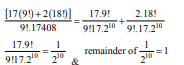Hence, the Remainder of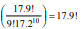2.18! = 2.18.17.16.15.14.13.12.11.10.9!

In 18, 16, 14, 12, 10 the number of 2s are 1, 4, 1, 2 & 1, respectively.

So 2.18! = 2.18.17.16.15.14.13.12 11.10. 9!

= 210.17k.9! = Where k is an integer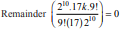So the required remainder is 17.9! + 0 = 17.9!.

16. If X is a natural number & X < 100 then the number of values of X for which 18X + 2 & 12X + 1 are relatively prime?
Solution:
If N divides both 18X + 2, 12X + 1 then their difference 6X + 1 will also be divisible by N & difference of 12X + 1 & 6X + 1 i.e. 6X will also be divisible by N. If 6X is divisible by N then N can also divide 12X. It means N divides both 12X, 12X + 1. Since 12X and 12X + 1 both are consecutive numbers so N = 1. So the given numbers are relatively prime for all values of X i.e. X would have 99 values.

17. P is a natural number of at least 6 digits and its leftmost digit is 7. When this leftmost digit is removed from P, the number thus obtained is found to be 1/21 times of P. What is the product of the all the nonzero digits of P.
(a) 126
(b) 105
(c) 60
(d) 72
Correct option: B
Solution:

Let P = 7 x 10⁵⁺ⁿ + k where n. K are whole numbers.
After removal of the leftmost digit the new number will be k.
According to the question:
7 x 10⁵ x 10ⁿ + k = 21k
7 x 10⁵ x 10ⁿ = 20k
k = (7 x 10⁵ x 10ⁿ) / 20
k = 35000 x 10ⁿ
P = 735000  x 10ⁿ
The required product = 7 x 3 x 5 = 105

18. X! is completely divisible by1151 but not by 1152. What is the sum of digits of largest such number X?
Solution:

X! is completely divisible by 1151. So the value of X should be less than 11 x 51 = 561 Highest power of 11 in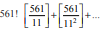= 51 + 4 = 55
If we subtract 11 x 3 = 33 from 561 we get 561 - 33 = 528 Highest power of 11 in 528! is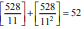Highest power of 11 in 528 - 1 = 527! is =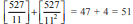So the required number is 527.
Sum of the digits = 5 + 2 + 7 = 14.

19. Find the lowest of three numbers as described: If the cube of the first number exceeds their product by 2, the cube of the second number is smaller than their product by 3, and the cube of the third number exceeds their product by 3.
(a) 31/3
(b) 91/3
(c) 2
(d) Any of these
(e) None of these
Correct option: D
Solution:
Let the product of the numbers be p. Then the cubes of the numbers are: p − 3, p + 2 and p + 3. The numbers (using the options) can be found to be 31/3; 91/3& 2.

20. How many pairs of natural numbers are there the difference of whose squares is 45?
(a) 1
(b) 2
(c) 3
(d) 4
Correct option: C
Solution:
(x − y)(x + y) = 45. Working through factor pairs of 45,we get 15 × 3; 45 × 1 & 9 × 5 as the three factor pairs here. The numbers are 9 &6; 22 &23; 7&2.

21. Find the last two digits of: 15 x 37 x 63 x 51 x 97 x 17.
(a) 35
(b) 45
(c) 55
(d) 85
Correct option:  A
Solution:

15 x 37 x 63 x 5 1 x 97 x 17 on division by 100 would give us a remainder that would be equal to its' last 2 digits. First we can divide the numerator and the denominator by 5 to get the expression: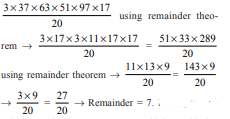Hence, the required remainder = 7 x 5 = 35, which would also be the last two digits of the given number.

22. Find the sum of all two-digit numbers that give a remainder of 3 when they are divided by 7.
(a) 686
(b) 676
(c) 666
(d) 656
Correct option: B
Solution:
The required numbers would be numbers in the Arithmetic Progression 10,17,24…94. The sum of this series would be given as n × Average = 13 × 104/2 = 676

23. How many times would 1 be used while writing all the natural numbers from 8 to 127 in the Binary number system?
(a) 212
(b) 218
(c) 424
(d) 436
Correct option: D
Solution:
We need to look at writing the binary number system from 8 =(1000). to 127 =(1111111), There are 64 7- digit numbers in Binary system are from 1000000 to 1111111. There are six digits after the leftmost 1. Each of these 6 digits can be filled by cither 0 or 1 and both are equally probable in any position So the number of 1 from 1000000 to 1111111 = 64 + 64 x 1/2 x 6 = 64 + 192 = 256

There are 32 6- digit numbers in Binary system are from 100000 to 111111. There are five digits after the leftmost 1. Each of these 5 digits can be filled by cither 0 or 1 and both are equally probable in any position So the number of I from 100000 to 111111 = 32 + 32 x 1/2 x 5 = 32 + 80 = 112.

Similarly, from 10000 to 11111 there are 16+ 16/2 x 4 = 16 + 32 = 48.
Similarly, from 1000 to 1111 there are 8 + 8 x 1/2 x 3 = 20
So total 1's = 20 + 48 + 112 + 256 = 436.

24. The sum of the digits of a three-digit number is 12. If we subtract 495 from the number consisting of the same digits written in reverse order, we shall get the required number. Find that three-digit number if the sum of all pairwise products of the digits constituting that number is 41.
(a) 156
(b) 237
(c) 197
(d) Both (a) and (b)
Correct option: D
Solution: Checking the options for the conditions, you would realise that both the values 156 and 237 satisfy the conditions of the problem. Note: Learning point from this question- The difference between a 3 digit number ‘abc’ and the 3 digit number ‘cba’ got by reversing the digits of abc, would be 99 × |a − c|.

25. A three-digit positive integer abc is such that a2 + b2 + c2 = 74. a is equal to the doubled sum of the digits in the tens and units places. Find the number if it is known that the difference between that number and the number written by the same digits in the reverse order is 495.
(a) 813
(b) 831
(c) 613
(d) 713
Correct option: A
Solution:
This can be easily checked through the options. However, if the options were not present, you could still do this by working out the sum of squares of the digits to be 74 (the only possible combination for three squares to add up to 74 would be 82 + 12 + 32 & 72 + 42 + 32.) Amongst these, we can select 8,1,3 as the digits since we also need the reversal of the digits to give us a difference of 495.

Offer running on EduRev: Apply code STAYHOME200 to get INR 200 off on our premium plan EduRev Infinity!

,

,

,

,

,

,

,

,

,

,

,

,

,

,

,

,

,

,

,

,

,

;